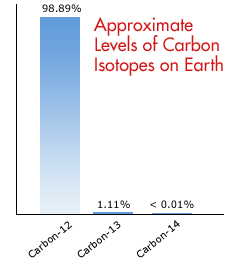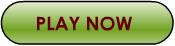# Isotopes, a Weighty Matter

 Chapter Pages ------------- A Weighty Matter Activity: Isotopes QuizTool: Interactive Periodic TableWhat is an Isotope?
An isotope is one particular form an atom of an element might take. Just as people may be thin, medium weight, or heavy, isotopes vary according to their weight. You will remember from the last chapter that the mass of an atom is determined by adding the number of protons to the number of neutrons in the nucleus. Since all the atoms of an element must have the same number of protons, the only thing that can vary to cause this change is the number of neutrons. This means that a "skinny" isotope would have a low number of neutrons. The "heavyweight" isotope would have the most neutrons. For example, there are three basic isotopes (forms) of carbon. The most common form has six protons, six electrons, and six neutrons. It is known as carbon-12, to designate the mass of the six protons plus the six neutrons. This is the skinny form. There is an extremely rare isotope of carbon known as carbon-13. This form has six protons, six electrons, and seven neutrons. It is the medium-weight form. Therefore, its atomic mass number would be 13. The least common, radioactive form of carbon is carbon-14. Carbon-14 contains six protons, six electrons, and eight neutrons per atom. This is the heavyweight form.

Atomic Mass and Isotopes
The atomic mass of carbon is listed as 12.011 atomic mass units per atom. This means that the vast majority of all carbon atoms have only six neutrons in their nucleus, but a small percentage of all carbon atoms may have seven or eight neutrons in their nucleus. To understand this mass number and its usefulness, we must first look at what is meant by "relative" mass. Obviously an atom is way too small for anyone to be able to accurately weigh one. There are no scales in the world sensitive enough to accomplish this. So scientists have come up with another method for measuring mass. They use the concept of a specific quantity, known as a mole, to compare the mass of different elements. One mole of any substance is 6.023 X 1023 atoms or molecules of that substance. In this way, moles are a counting unit kind of like dozens. Just as there are 12 items in a dozen of anything, there are 6.023 x 1023 items in a mole.

There is yet another way to define what a mole is that enables us to find out how many moles we have without having to count each individual atom or molecule. By definition, a mole of any element is the amount of that element in the gaseous state at standard temperature and pressure occupying a volume of 22.4 liters. Being able to take a container of the gaseous material and compare it to another container the same size under the same conditions of temperature and pressure allows us to compare the masses of the two elements. (This is only possible because scientists consider the volume of a gas particle so small as to be unimportant.)For example, if we have a box of 12 golf balls and an identical size box of 12 ping-pong balls, we can figure out how much the mass of one golf ball is compared to a ping-pong ball. This would be its relative mass. By massing the box of golf balls and then massing the box of ping-pong balls, we will be able to set up a ratio. Since ping-pong balls are so much less massive than golf balls, we will put the lower mass is the divisor to find that the golf ball is about 100 times more massive than a ping-pong ball. (This is a rough estimate and not necessarily accurate.) That would mean that if the ping-pong ball is our standard of comparison, it would have a mass value of 1, and the golf ball would have a mass value of 100.What's "massing"? Is that the same as "weighing"? In this case, massing could be generally equated to weighing something, but in hard scientific terms, weight refers to the gravitational attraction of one body for another. This means that your weight is different on the moon from what it would be on Earth, but you have not lost any mass. Mass is the amount of "stuff" present. This is not to be confused with volume!In the same general way, scientists have chosen the form of carbon that has six protons, six neutrons, and six electrons (carbon-12) as the standard for measuring all elements' mass. The number with the decimals in it in the element box on the periodic table is the relative mass (compared to 1/12 the mass of one atom of carbon-12) of the element according to the abundance of isotopes of the element.

Calculating Mass
Let's look at how to calculate this average relative atomic mass for an imaginary element -- Utopium. Utopium exists with three different isotopes. All forms of Utopium (Ut) have 150 protons and 150 electrons in their atoms. The most common form has 150 neutrons in its nucleus. The mass of this form is 300, making this isotope Ut-300. The other two forms have 155 and 160 neutrons in their nuclei, making them Ut-305 and Ut-310, respectively. As it happens, 83 of every 100 atoms of Utopium exist as Ut-300. The Ut-305 form makes up 12 atoms of every 100, leaving 5 atoms of Ut-310 in every 100. To calculate the average relative mass of Utopium, you must multiply the mass of the isotope times its percentage of relative abundance, and then add the answers. Below is the formula as a straight math problem:

 Ut-300 (300)(.83) = 249.00 Ut-305 (305)(.12) = 36.60 Ut-310 (310)(.05) = 15.00300.60 atomic mass units is the average relative mass

This means the average atom of Utopium is just a fraction more than 300 times as massive as 1/12 of one atom of carbon.

Think you know how to calculate an atom's average relative mass? Test your skills nowNext: Isotopes Quiz